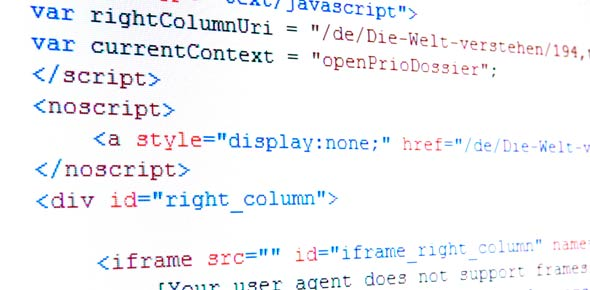# F129 Introduction To Programming Test 1

Approved & Edited by ProProfs Editorial Team
The editorial team at ProProfs Quizzes consists of a select group of subject experts, trivia writers, and quiz masters who have authored over 10,000 quizzes taken by more than 100 million users. This team includes our in-house seasoned quiz moderators and subject matter experts. Our editorial experts, spread across the world, are rigorously trained using our comprehensive guidelines to ensure that you receive the highest quality quizzes.
| By Xenonii
X
Xenonii
Community Contributor
Quizzes Created: 4 | Total Attempts: 968
Questions: 10 | Attempts: 266SettingsThis test will ask you questions up to Chapter 6 of the study guide

• 1.

### The result of this expression 3 + 5 * 2 + 4 is

• A.

20

• B.

64

• C.

17

• D.

None of the above

C. 17
Explanation
A computer language uses operator precedence (hierarchy) similar to what we use in maths (BODMAS).

Rate this question:

• 2.

• 3.

### Call-by-value is

• A.

When you declare a variable of some certain data type

• B.

When a variable's value is copied into a parameter when calling some method

• C.

When you refer to a variable

• D.

When you assign a value to a variable

B. When a variable's value is copied into a parameter when calling some method
Explanation
You can pass a variable to a method using call-by-value or call-by-reference. Call-by-value is simply copied into the parameter, but call-by-reference is when the actual variable is being referenced by the method you are calling. Refer to the revision sheet 2 for more information.

Rate this question:

• 4.

• 5.

### The AND and OR logical operators take two operands one on each side. What are the data types of these operands?

• A.

String

• B.

Integer

• C.

Character

• D.

Boolean

• E.

None of the above

D. Boolean
Explanation
The operands are some boolean expression.E.g. if you want to check some mark if its greater or equal to 0 and smaller or equal to 100 you would do something like this: if mark >= 0 AND mark = 0 gives true or false, and mark =0 AND mark

Rate this question:

• 6.

### The data type of the following expression squareroot(14) is

• A.

Integer

• B.

String

• C.

Real

• D.

3.741

C. Real
Explanation
Reals are those numbers with a decimal point

Rate this question:

• 7.

### What is the data type of "hello "+name, where name is some variable storing the user's name

• A.

String

• B.

Character

• C.

Integer

• D.

None of the above

A. String
Explanation
The data type of "hello "+name would be a string because when we concatenate the string "hello " with the variable name, the result will be a string. In programming, when we combine two strings together, the result is always a new string. Therefore, the correct answer is String.

Rate this question:

• 8.

### How would you end a module which gives back the addition of 3 numbers. The module so far looks like thisCalculateSum(Integer a, Integer b, Integer c)   Integer sum = a + b + c

• A.

End

• B.

Exit

• C.

Return sum

• D.

None of the above

C. Return sum
Explanation
The correct answer is "Return sum" because the module is designed to calculate the sum of three numbers and the result is stored in the variable "sum". To end the module and provide the calculated sum as the output, the "Return" statement is used. This will return the value of the "sum" variable and terminate the execution of the module.

Rate this question:

• 9.

### How would you call the module from the Control module to give you back the result of the sum, using the module mentioned in the previous question. So far the Control module looks like this:Control   Print "Give me three numbers"   Integer x   Enter x   Integer y   Enter y   Integer z   Enter z

• A.

Process CalculateSum(x,y,z)

• B.

Process CalculateSum(a, b, c)

• C.

Process CalculateSum(*x,*y,*z)

• D.

Process CalculateSum(*a, *b, *c)

• E.

None of the above

A. Process CalculateSum(x,y,z)
Explanation
The correct answer is "Process CalculateSum(x,y,z)" because this line of code is calling the CalculateSum module and passing the variables x, y, and z as arguments. This means that the Control module is instructing the CalculateSum module to perform the calculation and return the result. The asterisks (*) in the other options are not valid syntax and do not correctly call the CalculateSum module.

Rate this question:

• 10.

### Write a small program that asks the user for two numbers and you need to display back the biggest number of the two numbers that he gives you. Ideally you would use modules. Remember that you always have the Control module, then we usually have three other modules, one which caters for the input, another for the calculation, and another for the output. Also remember that in pseudo code you use Print to output on the user's screen, and Enter to wait for input from the user's keyboard. Also remember to declare the variable data type before using a variable.

Related TopicsBack to top